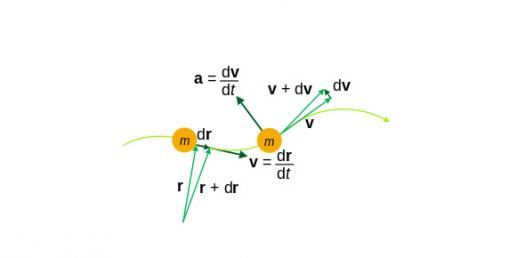# How Much Do You Know About Acceleration?

10 Questions | Total Attempts: 1095SettingsQuiz for Period 2 Computer based science class. Quiz is from the Holt Science Spectrum.

• 1.
Acceleration can mean
• A.

Speeding up.

• B.

Slowing down.

• C.

Changing direction.

• D.

All of the above

• 2.
Centripetal acceleration occurs because an object is
• A.

Speeding up.

• B.

Slowing down.

• C.

Changing direction.

• D.

None of the above

• 3.
Which of the following is constant during uniform circular motion?
• A.

Velocity

• B.

Speed

• C.

Displacement

• D.

Direction

• 4.
The average acceleration of a car that goes from 0 m/s to 25 m/s in 8.0 s is.
• A.

0.32 m/s

• B.

0.32 m/s^2

• C.

3.1 m/s

• D.

3.1 m/s^2

• 5.
A cyclist is traveling at an initial speed of 10.0 m/s.  She accelerates at a rate of 0.500 m/s^2.  Find her final speed after 9.0 s.
• A.

14.5 m/s

• B.

14.5 m/s^2

• C.

5.5 m/s

• D.

5.5 m/s^2

• 6.
Peter runs in a constant direction on a straight road.  The velocity-time graph of Peter's motion shows a straight line with a negative slope.  What does the line tell you about Peter's speed?
• A.

It is increasing.

• B.

It is decreasing.

• C.

It is increasing, then decreasing.

• D.

It is constant.

• 7.
When velocity changes the same amount over each time interval, acceleration is
• A.

Zero.

• B.

Constant.

• C.

Positive.

• D.

Negative.

• 8.
On a velocity-time graph, what shows the value of acceleration?
• A.

The slope of the line

• B.

The x-axis

• C.

The y-axis

• D.

The final velocity

• 9.
A cheetah can accelerate at up to 6.0 m/s^2.  How long does it take for a cheeta to speed up from 10.5 m/s to 12.2 m/s?
• A.

0.28 s

• B.

1.7 s

• C.

3.5 s

• D.

10 s

• 10.
What does it mean when a change in velocity is negative?
• A.

The object is speeding up.

• B.

The object is changing direction.

• C.

The object is slowing down.

• D.

The object is falling down.

Related TopicsBack to top